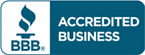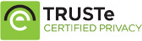# Test bank for Statistics for Business & Economics, 12th Edition by David R. Anderson

\$30.00

Statistics for Business & Economics, 12th Edition by David R. Anderson Test bankCategories: ,

## Description

Test bank for Statistics for Business & Economics, 12th Edition by David R. Anderson

1. Data and Statistics.
2. Descriptive Statistics: Tabular and Graphical Displays.
3. Descriptive Statistics: Numerical Measures.
4. Introduction to Probability.
5. Discrete Probability Distributions.
6. Continuous Probability Distributions.
7. Sampling and Sampling Distributions.
8. Interval Estimation.
9. Hypothesis Tests.
10. Inference about Means and Proportions with Two Populations.
11. Inferences about Population Variances.
12. Comparing Multiple Proportions, Test of Independence and Goodness of Fit.
13. Experimental Design and Analysis of Variance.
14. Simple Linear Regression.
15. Multiple Regression.
16. Regression Analysis: Model Building.
17. Time Series Analysis and Forecasting.
18. Nonparametric Methods.
19. Statistical Methods for Quality Control.
20. Index Numbers.
21. Decision Analysis.
22. Sample Survey (online).
Appendix A: References and Bibliography.
Appendix B: Tables.
Appendix C: Summation Notation.
Appendix D: Self-Test Solutions and Answers to Even –Numbered Exercises.
Appendix E: Microsoft Excel 2013 and Tools for Statistical Analysis.
Appendix F: Computing p-Values Using Minitab and Excel.

## Reviews

There are no reviews yet.